## Selina Concise Mathematics Class 10 ICSE Solutions Chapter 22 Heights and Distances Ex 22B

These Solutions are part of Selina Concise Mathematics Class 10 ICSE Solutions. Here we have given Selina Concise Mathematics Class 10 ICSE Solutions Chapter 22 Heights and Distances Ex 22B

Other Exercises

Question 1.
In the figure given below, it is given that AB is perpendicular to BD and is of length X metres. DC = 30 m. ∠ADB = 30° and ∠ACB = 45°. Without using tables, find X.
Solution: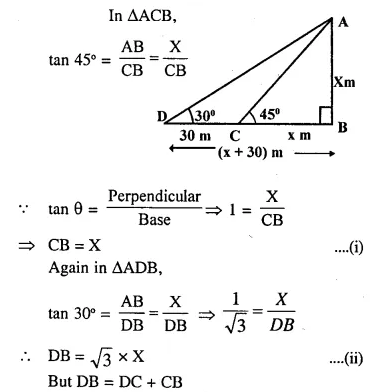Question 2.
Find the height of a tree when it is found that on walking away from it 20m, in a horizontal line through its base, the elevation of its top Changes from 60° to 30°.
Solution:
Let AB be the tree and its height be x DC = 20 m.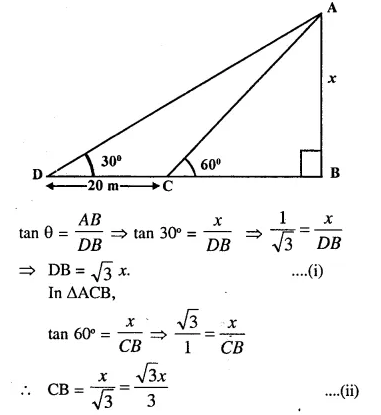Question 3.
Find the height of a building, when it is found that on walking towards it 40 m in a horizontal line through its base the angular elevation of its top changes from 30° to 45°.
Solution:
Let AB be the building and its
height be x and DC =40m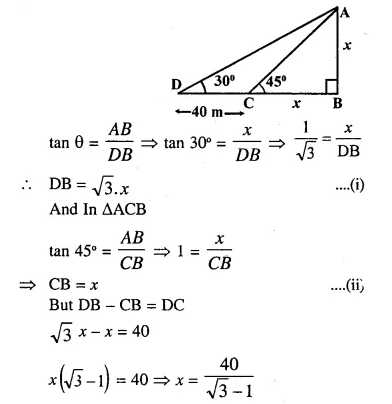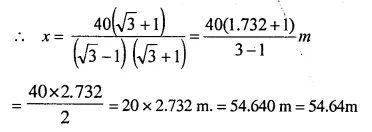Question 4.
From the top of a light house 100 m high, the angles of depression of two ships are observed as 48° and 36° respectively. Find the distance between the two ships (in the nearest metre) if :
(i) the ships are on the same side of the light house,
(ii) the ships are on the opposite sides of the light house.
Solution:
In right angled ∆ABD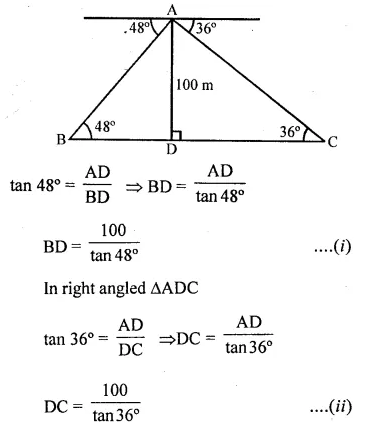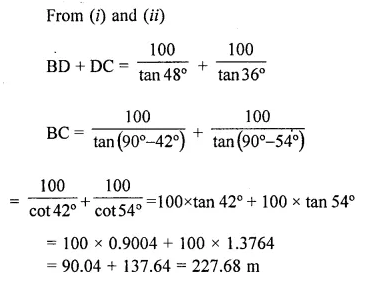Question 5.
Two pillars of equal heights stand 011 either side of a roadway, which is 150m wide. At a point in the roadway between the pillars the elevations of the tops of the pillars are 60° and 30”; find the height of the pillars and the position of the point.
Solution:
Let AB and CD be the two pillars which stand on either side of a road BD, then BD = 150m
Let AB = CD = h
Let P be the point on the road such that the angles of elevation from P to the top of the pillars are 60° and 30° respectively.
Let BP = x then PD = 150 – x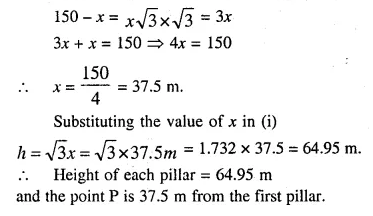Question 6.
From the figure given below, calculate the length of CD.Solution: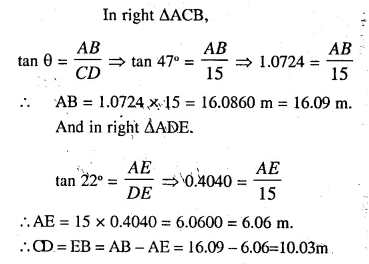Question 7.
The angle of elevation of the top of a tower is observed to be 60°. At a point 30 m vertically above the first point of observation, the elevation is found to be 45°. Find :
(i) the height of the tower,
(ii) its horizontal distance from the points of observation.
Solution:
Let height of tower = x i.e. AB = x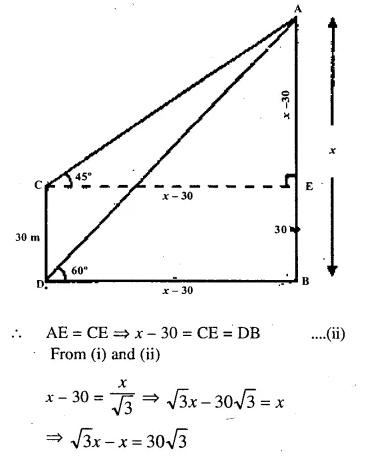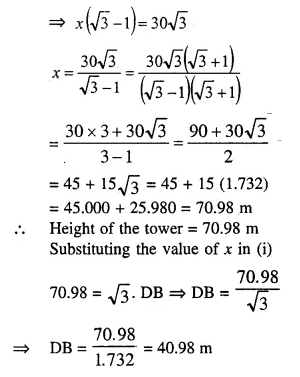Question 8.
From the top of a cliff. 60 metre high, the angles of depression of the top and bottom of a tower are observed to be 30° and 60°. Find the height of the tower.
Solution:
Height of the cliff AB = 60m
Let the height of tower = x
Draw TR $$\parallel$$ SB. meeting AB in R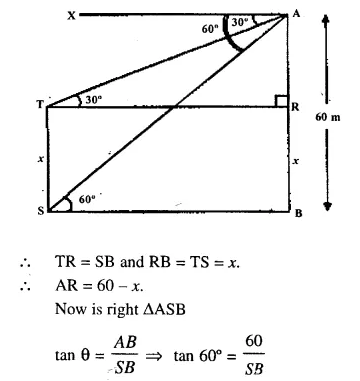Question 9.
A man on a cliff observes a boat, at an angle of depression 30°, which is sailing towards the shore to the point immediately beneath him. Three minutes later the angle of depression of the boat is found to be 60°. Assuming that the boat sails at a uniform speed, determine :
(i) how much more time it will take to reach the shore.
(ii) the speed of the boat in metre per second, if the height of the cliff is 500 m.
Solution:
Height of cliff = 500 m.
In right ∆ACD,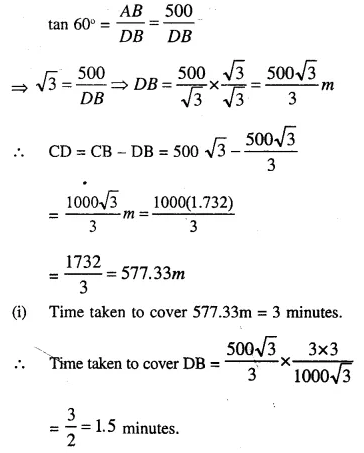Question 10.
A man in a boat rowing away from a light house 150m high, takes 2 minutes to change the angle of elevation of the top of the lighthouse from 60° to 45°. Find the speed of the boat.
Solution:
Height of the lighthouse AB = 150m
Now is right ∆ACB,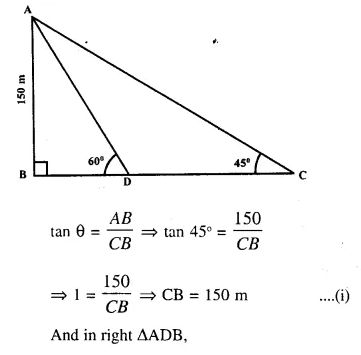Question 11.
A person standing on the bank of a river observes that the angle of elevation of the top of a tree standing on the opposite bank is 60°. When he moves 40 m away from the bank, he finds the angle of elevation to be 30°. Find :
(i) the height of the tree, correct to 2 decimal places,
(ii) the width of the river.
Solution:
TR is the height of tree and RQ is the width of the river.
Let TR = x
Now in right ∆TQR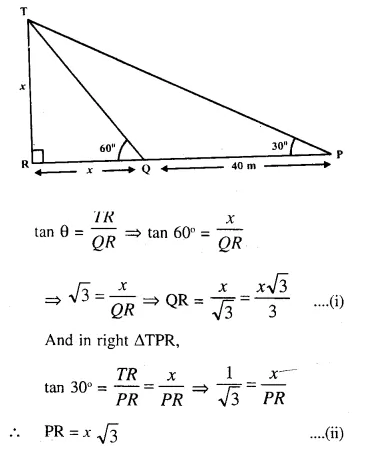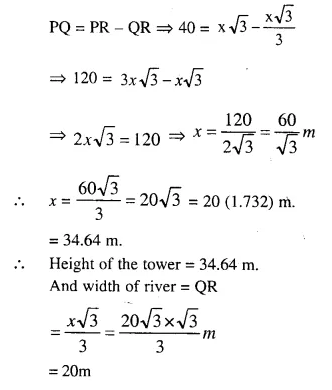Question 12.
The horizontal distance between two towers is 75 m and the angular depression of the top of the first tower as seen from the top of the second, which is 160m high, is 45°. Find the height of the first tower.
Solution: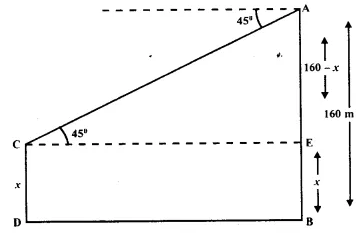Let the height of first tower CD = x
and height of second tower AB = 160 m
Distance between them DB = 75 m.
In right ∆ACE,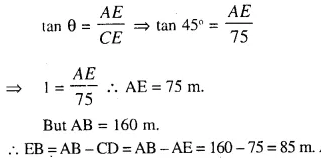Question 13.
The length of the shadow of a tower standing on level plane is found to be 2y metres longer when the sun’s altitude is 30″ than when it was
45°. Prove that the height of the tower is y ($$\sqrt { 3 }$$ +1) metres.
Solution:
Let AB be the tower and AB = x
Distance CD = 2yQuestion 14.
An aeroplane flying horizontally 1 km above the ground and going away from the observer is observed at an elevation of 60°. After 10 seconds, its elevation is observed to be 30°, find the uniform speed of the aeroplane in km per hour.
Solution:
Height of aeroplane = 1 km = 1000 m.
In right ∆ACB,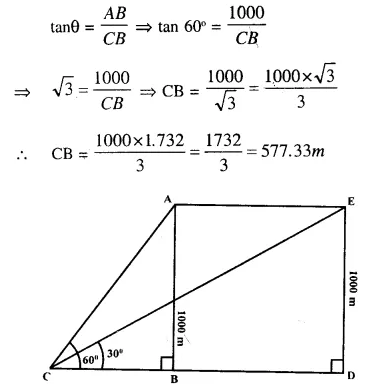Question 15.
From the top of a hill, the angles of depression of two consecutive kilometre stones, due east, are found to be 30° and 45° respectively. Find the distance of the two stones from the foot of the hill. (2007)
Solution: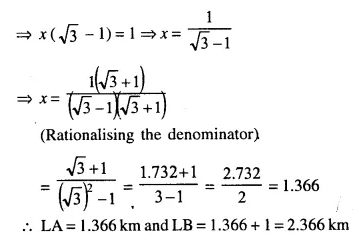Hope given Selina Concise Mathematics Class 10 ICSE Solutions Chapter 22 Heights and Distances Ex 22B are helpful to complete your math homework.

If you have any doubts, please comment below. Learn Insta try to provide online math tutoring for you.